## 682 Reputation

3 years, 220 days
changsha, China

## Can converting mathematical expressions ...

Maple 2022

I have the following expression:

```q1:=2.800000000*10^(-30)*a+2.7800000000*10^(-29)*b+2.7800000000*10^(-31)+3.0*10^(-21)+2.*10^(-32)*c+3.*10^(-30)*d;
```

I saved it as a string, but the format seems to have changed.

`q2:=convert(q1,string)`

Can I output it as follows:

`"2.800000000*10^(-30)*a+2.7800000000*10^(-29)*b+2.7800000000*10^(-31)+3.0*10^(-21)+2.*10^(-32)*c+3.*10^(-30)*d"`

## Is there a maple function comparable to ...

Maple 2022

Recently, I tried to write a function to get the lexicographic product of two graphs.

In  graph theory the lexicographic product G ∙ H of graphs G and H is a graph such that
• the vertex set of G ∙ H is the cartesian product V(G) × V(H); and
• any two vertices (u,v) and (x,y) are adjacent in G ∙ H if and only if either u is adjacent with x in G or u = x and v is adjacent with y in H.

I'm trying to write this function as defined after making sure it doesn't exist in maple. I saw a similar post by mathematica stack.

There are many answers in the post, the most interesting to me is the following code, which follows the definition of lexicographic product.

``````lexicographicProduct[g1_?UndirectedGraphQ, g2_?UndirectedGraphQ, opt : OptionsPattern[]] :=
RelationGraph[
(* two nodes are connected if their corresponding nodes in the first graph are connected *)
EdgeQ[g1, First[#1] \[UndirectedEdge] First[#2]] ||
(* or their corresponding nodes in the first graph are the same and their corresponding nodes in the second graph are connected *)
(First[#1] === First[#2] && EdgeQ[g2, Last[#1] \[UndirectedEdge] Last[#2]]) &,

(* the vertices are the cartesian product of the two vertex sets *)
Tuples[{VertexList[g1], VertexList[g2]}],

(* also allow setting graph options *)
opt
]

lexicographicProduct[CycleGraph, CycleGraph]``````

It utilizes the  function `RelationGraph` in Mathematica. I feel that this function is generic in nature. So here I would ask maple if they had a similar function.

Function `RelationGraph` is to generate a graph based on data and a binary relation.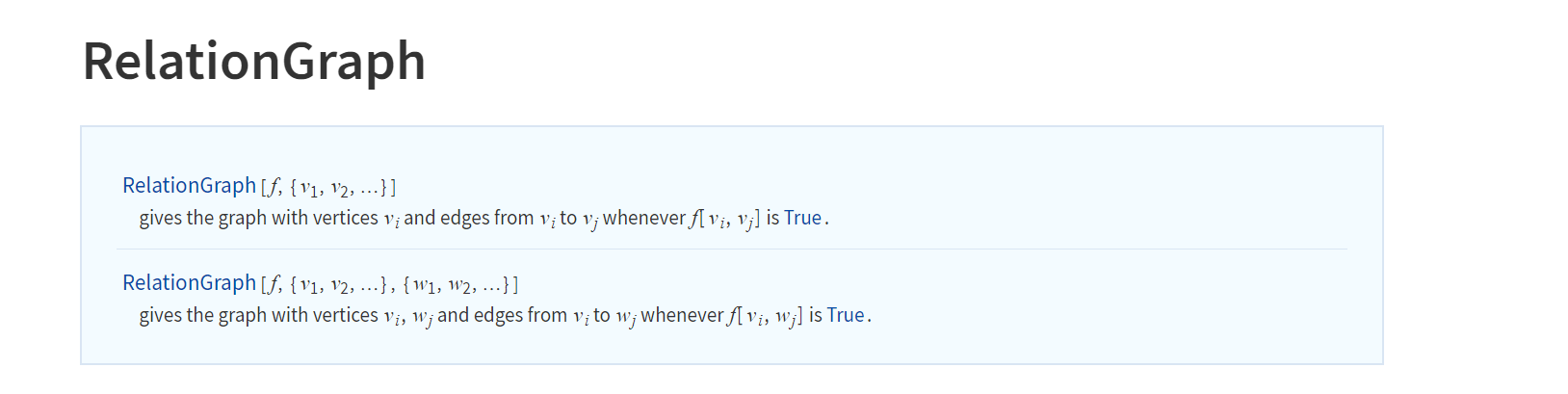For example, using `RelationGraph`  I  can get easily  the kth power Gk of an graph G which is another graph that has the same set of vertices, but in which two vertices are adjacent when their distance in G is at most k.

```Dis[g1_?UndirectedGraphQ, k_] :=
RelationGraph[
GraphDistance[g1, #1, #2] <= k && GraphDistance[g1, #1, #2] != 0 &,
VertexList[g1]]
Dis[PathGraph[Range], 2]
```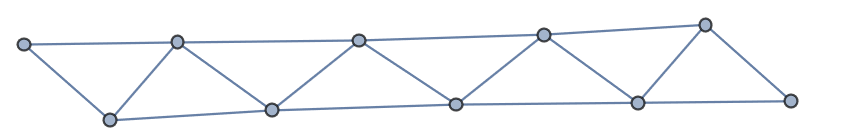If I use maple and do not use the built-in function `GraphPower`, I might deal with the following.

```with(GraphTheory):
with(SpecialGraphs):
graphpower:=proc(G,k):
local choo,edge,vex,g;
vex:=convert(Vertices(G),list);
choo:= choose(vex, 2):
g:= Graph(Vertices(G)):
for edge in choo do
if Distance(G, edge, edge)<=k  then
fi;
end do:
return g;
end proc:
s:=graphpower(PathGraph(10),2);DrawGraph(s)
```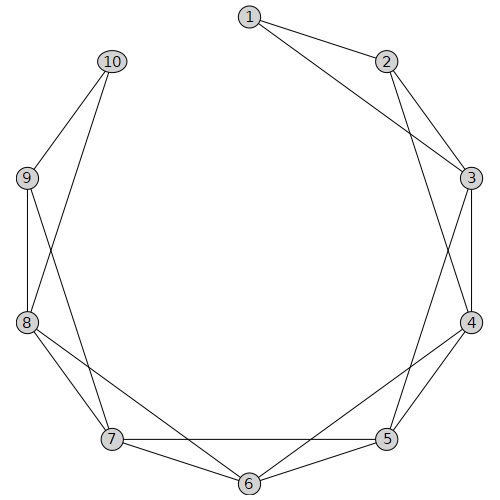I believe if the `RelationGraph` function can be  implemented in maple, the function `lexicographicProduct` would be easier to obtain.

## Can Maple's functions be packaged as exe...

Maple 2022

The problem comes from the link https://www.mapleprimes.com/questions/234398-Convert-Maple-Code-To-Python-#comment287424.

We know that when we compile a C or C ++ function, it generates an executable file.  Then we are free from source code.  For example. the function below returns a square matrix A where    "A[i, j]" is the distance from vertex i to vertex j in the graph G. My computer system is Windows.

```// C Program for Floyd Warshall Algorithm
#include <stdio.h>

// Number of vertices in the graph
#define V 4

/* Define Infinite as a large enough
value. This value will be used
for vertices not connected to each other */
#define INF 99999

// A function to print the solution matrix
void printSolution(int dist[][V]);

// Solves the all-pairs shortest path
// problem using Floyd Warshall algorithm
void floydWarshall (int graph[][V]) {
/* dist[][] will be the output matrix
that will finally have the shortest
distances between every pair of vertices */
int dist[V][V], i, j, k;

/* Initialize the solution matrix
same as input graph matrix. Or
we can say the initial values of
shortest distances are based
on shortest paths considering no
intermediate vertex. */
for (i = 0; i < V; i++)
for (j = 0; j < V; j++)
dist[i][j] = graph[i][j];

/* Add all vertices one by one to
the set of intermediate vertices.
---> Before start of an iteration, we
have shortest distances between all
pairs of vertices such that the shortest
distances consider only the
vertices in set {0, 1, 2, .. k-1} as
intermediate vertices.
----> After the end of an iteration,
vertex no. k is added to the set of
intermediate vertices and the set
becomes {0, 1, 2, .. k} */
for (k = 0; k < V; k++) {
// Pick all vertices as source one by one
for (i = 0; i < V; i++) {
// Pick all vertices as destination for the
// above picked source
for (j = 0; j < V; j++) {
// If vertex k is on the shortest path from
// i to j, then update the value of dist[i][j]
if (dist[i][k] + dist[k][j] < dist[i][j])
dist[i][j] = dist[i][k] + dist[k][j];
}
}
}

// Print the shortest distance matrix
printSolution(dist);
}

/* A utility function to print solution */
void printSolution(int dist[][V]) {
printf ("The following matrix shows the shortest distances"
" between every pair of vertices \n");
for (int i = 0; i < V; i++) {
for (int j = 0; j < V; j++) {
if (dist[i][j] == INF)
printf("%7s", "INF");
else
printf ("%7d", dist[i][j]);
}
printf("\n");
}
}

// driver program to test above function
int main() {
/* Let us create the following weighted graph
10
(0)------->(3)
|         /|\
5 |          |
|          | 1
\|/         |
(1)------->(2)
3           */
int graph[V][V] = { {0,   5,  INF, 10},
{INF, 0,   3, INF},
{INF, INF, 0,   1},
{INF, INF, INF, 0}
};

// Print the solution
floydWarshall(graph);
return 0;
}```

The above functions will be packaged as the disall.exe , and then we will move them anywhere in my computer and run it in Powershell.  We don't have to deal with the source code unless we want to change it.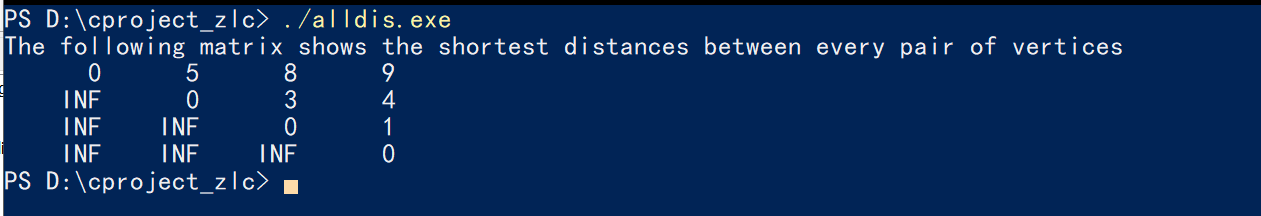I mean can Maple do something like that?

```with(GraphTheory);
G := Graph([1, 2, 3, 4, 5], {{1, 2}, {1, 3}, {1, 4}, {1, 5}, {2, 3}, {2, 5}, {3, 4}, {4, 5}});
AllPairsDistance(G);
```

For exmaple, can I package the above code snippet into an exe file?

## How to remove the mosaic of vertices?...

Maple 2022

I  generated some graphs via maple and would like to put them in my paper. So I am going to convert the following worksheet to pdf.

```with(GraphTheory):
Graphs:=[NonIsomorphicGraphs(6,8,output=graphs,outputform = graph)]:
num_g:=nops(Graphs):
num:=ceil((num_g)/5.):
M1:=Matrix (num,5,(i,j)->`if`((i-1)*5+j<=num_g, DrawGraph(Graphs[(i-1)*5+j],size=[250,250] ,overrideoptions ,showlabels=false,style=planar, stylesheet =  [
vertexcolor     = orange
,vertexfontcolor = black
,vertexborder    = false
,edgethickness   = 0.6
,edgecolor       = MidnightBlue
,vertexshape     =  "circle"
,vertexfont      = [Arial, 4],
vertexthickness=5], caption = cat(H__,5*(i-1)+j),captionfont=["ROMAN",7]),plot(x = 0 .. 1, axes = none))):
DocumentTools:-Tabulate (M1[1..5,.. ],widthmode=percentage ,width=80 , exterior =all) :
```

But there was a problem with the exported pdf. There was some mosaic stuff at the vertices of all those graphs. It was strange. (I want to reduce the size of vertices of the graphs in order not to look crowded.)Only when I insert the option vertexpadding and set a large enough size  of vertex (for this example, we set vertexpadding=7),  it won't go wrong. However, in fact, we often need make vertice‘s size smaller , especially when there are more vertices.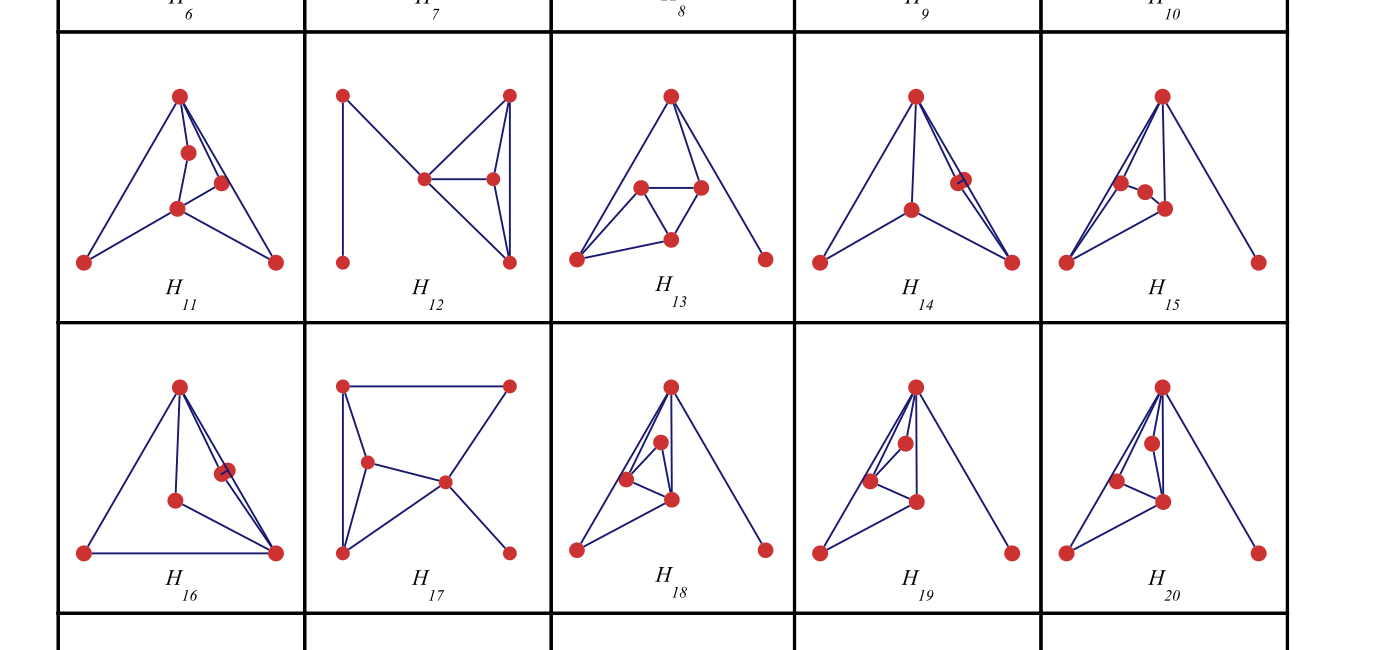## Can Maple import the graphs generated by...

Maple 2022

I've been asking a similar question：

How does Maple call external programs such as nauty?

Recently I used Mathematica to call these gadgets very succinctly, so I revisit the topic, and maybe Maple can do it as well, but I just didn't do it the right way.

• nauty and Traces are programs for computing automorphism groups of graphs and digraphs. There is a small suite of programs called gtools included in the package. For example, geng can generate non-isomorphic graphs very quickly.
• plantri and fullgen are programs for generation of certain types of planar graph.

We note that binary executables of above two programs for Windows are not officially available. Fortunately, I recently compiled them by cygwin.  Of course, other operating systems can make it easier to use them. See attached two compressed files of compiled nauty and plantri programs for Windows.

The official websites of the two programs are listed below.

So, I found that Mathematica works very well for running these programs by Import. We list the following two examples: first example is to get all non-isomorphic 10-order 2-partite connected graph, and the second example is to get all 14-order non-isomorphic  quadrilateral graphs (the planar graphs in which any face is 4-face).

```glist10 = Import["!D:/nauty27r3/geng -c -b 10", "Graph6"]; // AbsoluteTiming
Length[glist10]````g14 = Import["!D:/plantri52/plantri 14 -q -g ", "Graph6"] // AbsoluteTiming`I tried maple's Import or ImportGraph functions, but both failed.

```restart:
with(GraphTheory):
L:=ImportGraph("!D:/nauty27r3/geng -c -b 10 -g",output=list):
L2:=Import("!D:/nauty27r3/geng -c -b 10 -g"):
```

Error, invalid input: GraphTheory:-ImportGraph uses a 2nd argument, format (of type {string, symbol}), which is missing
Error, (in Import) must specify format for this input

The problem seems to be not recognizing compilations symbols " !".  I don't know if Maple can do this like mathematica.

 1 2 3 4 5 6 7Last Page 1 of 18
﻿